# Selina Solutions Concise Mathematics Class 6 Chapter 27: Quadrilateral

Selina Solutions Concise Mathematics Class 6 Chapter 27 Quadrilateral has accurate answers on fundamental concepts covered under this chapter. A quadrilateral is defined as a plane closed figure with four sides. Experts prepare the solutions with the intention to boost exam preparation of students. Those who find difficulty in solving questions can use these solutions, to speed up the problem solving abilities. For a better academic score, Selina Solutions Concise Mathematics Class 6 Chapter 27 Quadrilateral, PDF links are presented below with a free download option.

Chapter 27 deals with the method of finding the angle of a given set of quadrilaterals. The important formula and the method of solving problems are given in a descriptive manner, for a better conceptual knowledge among students.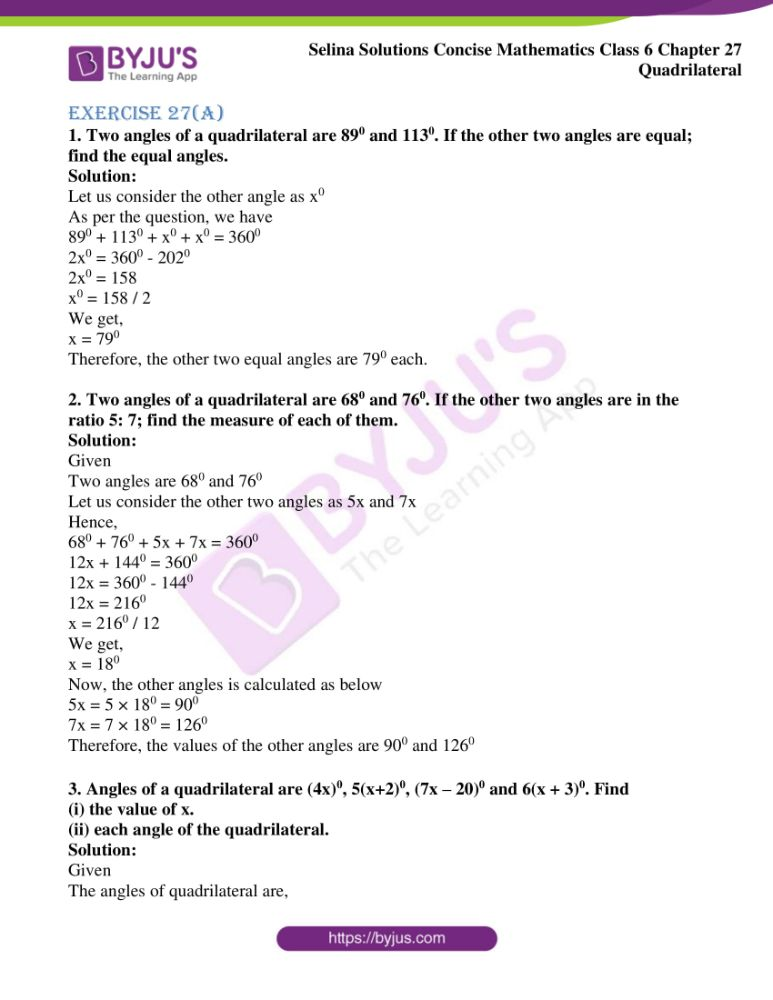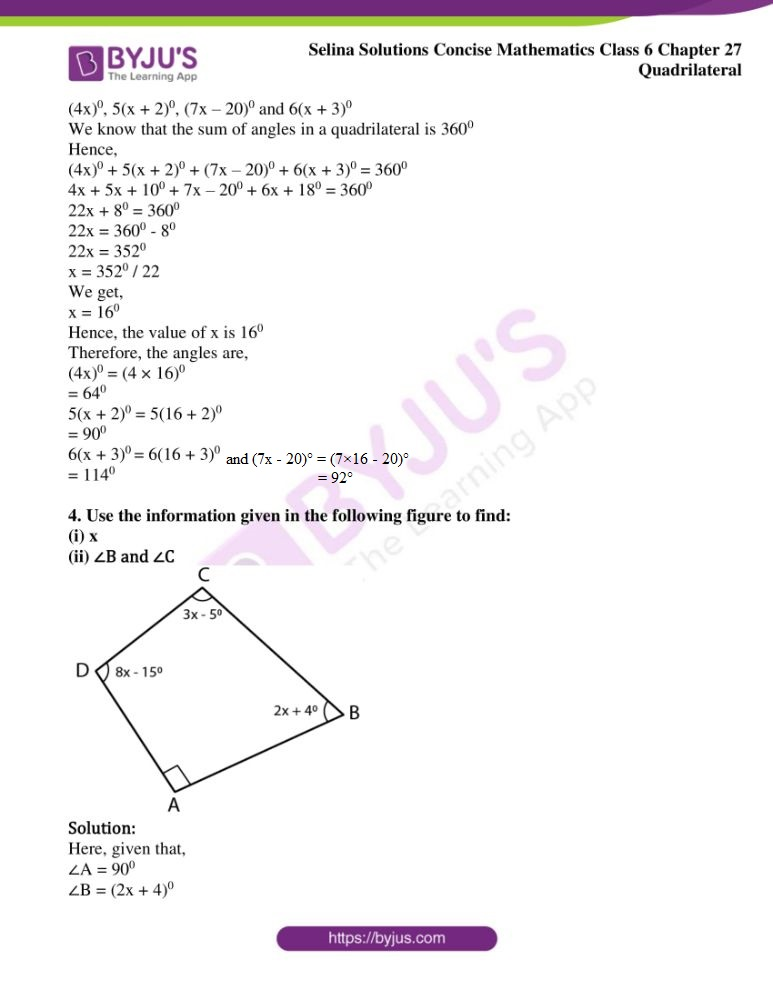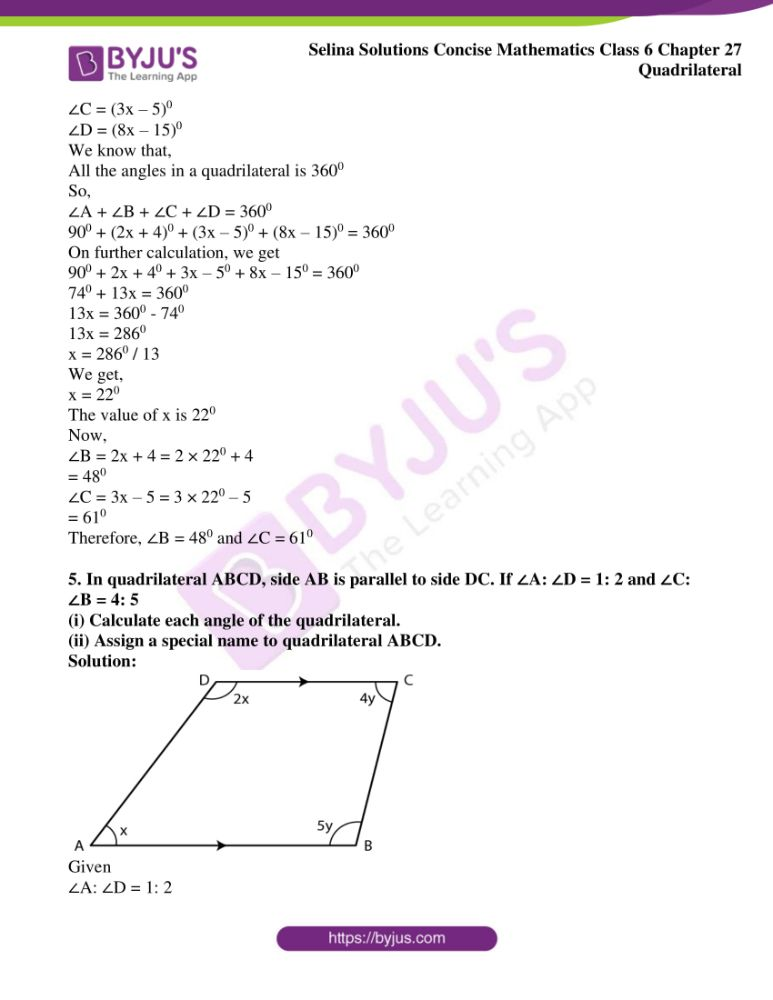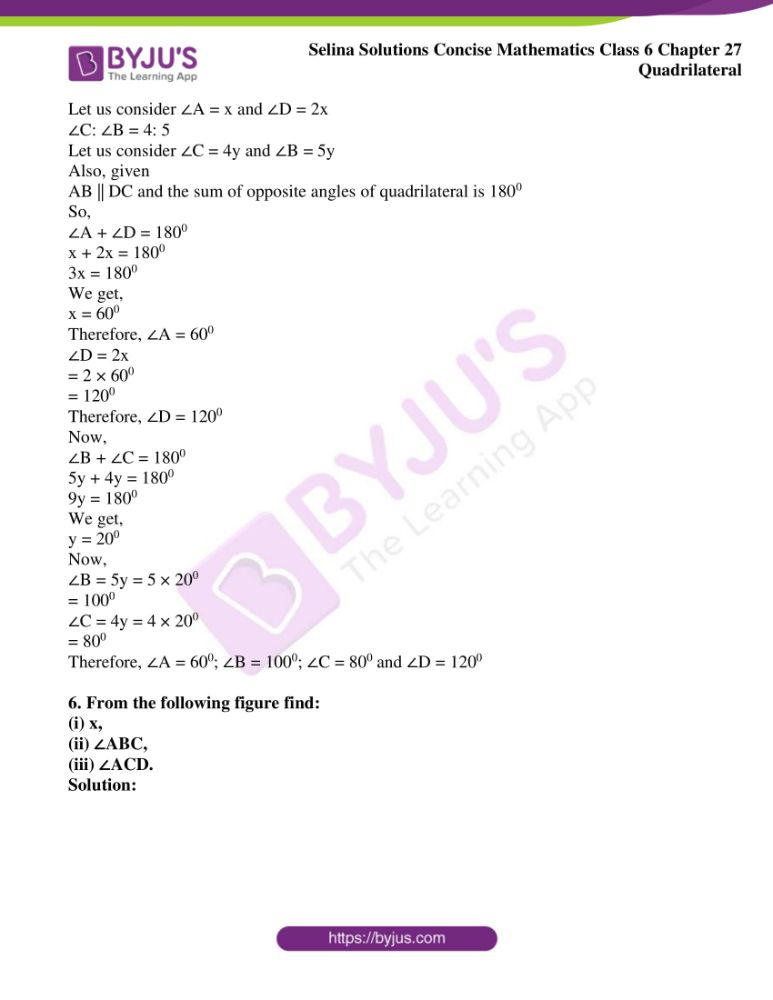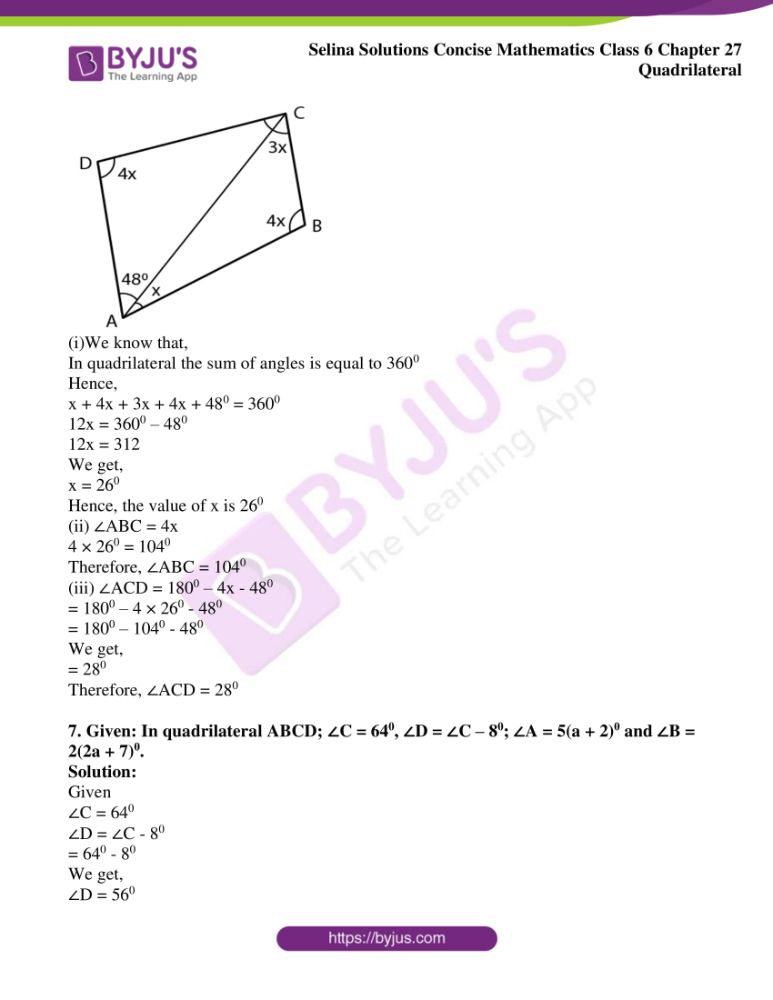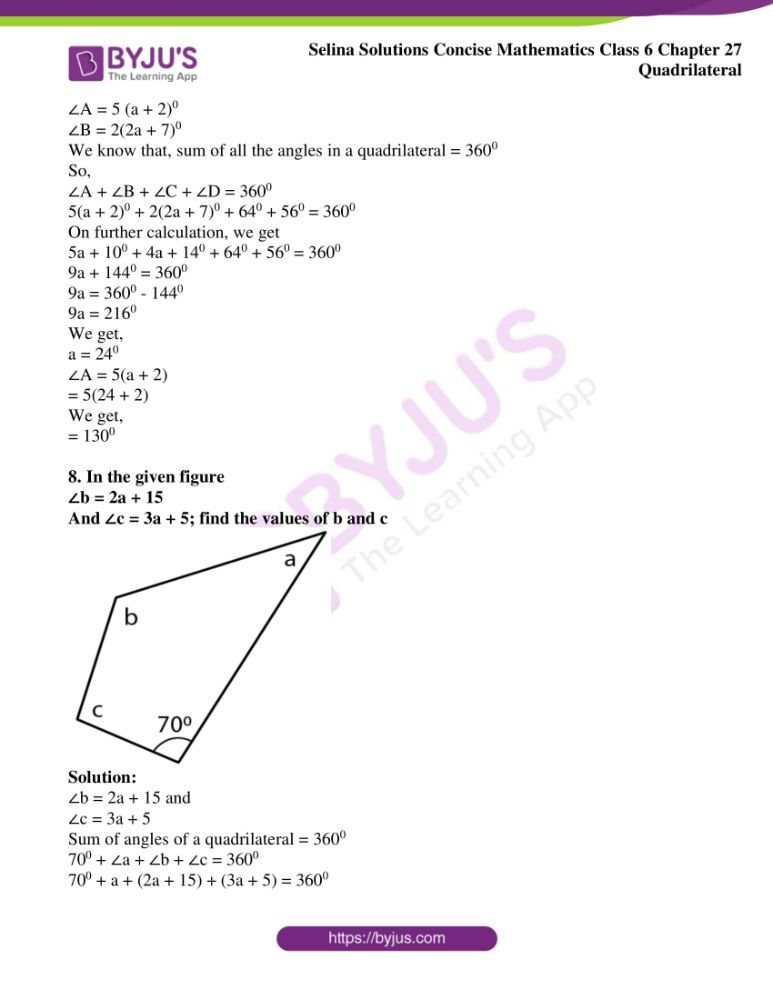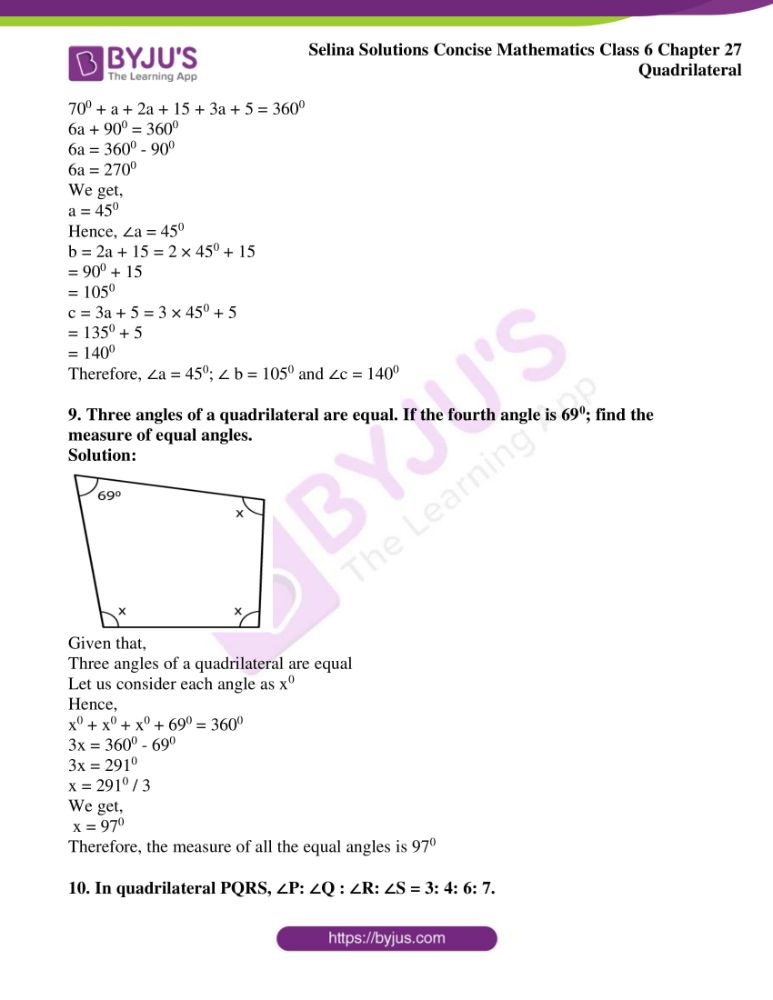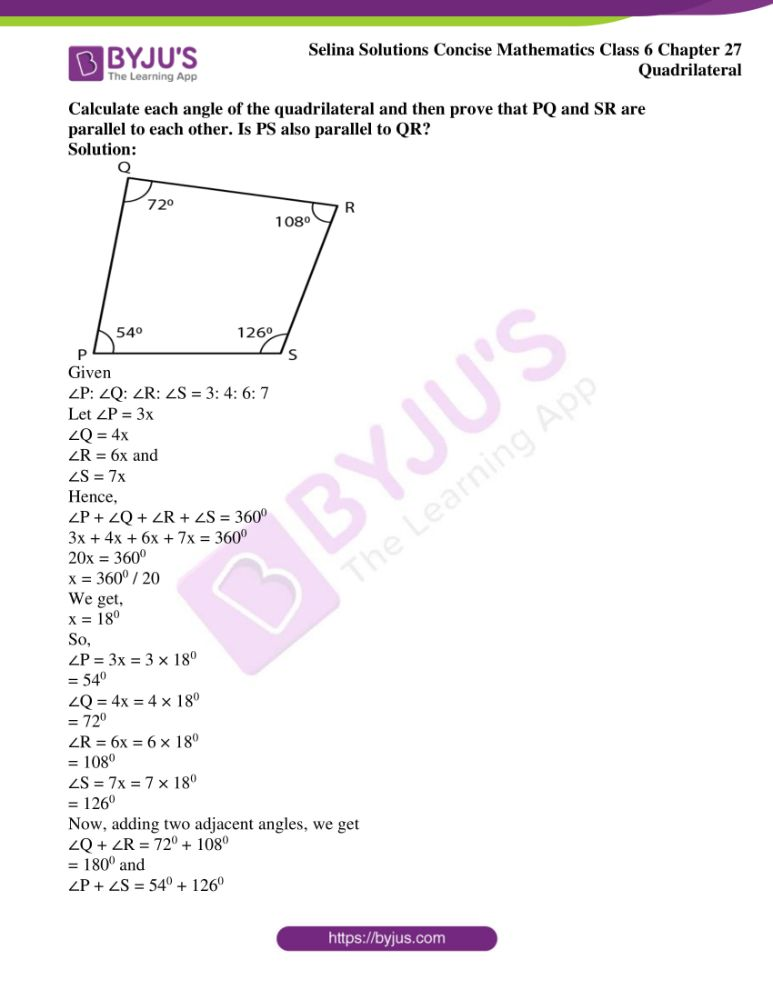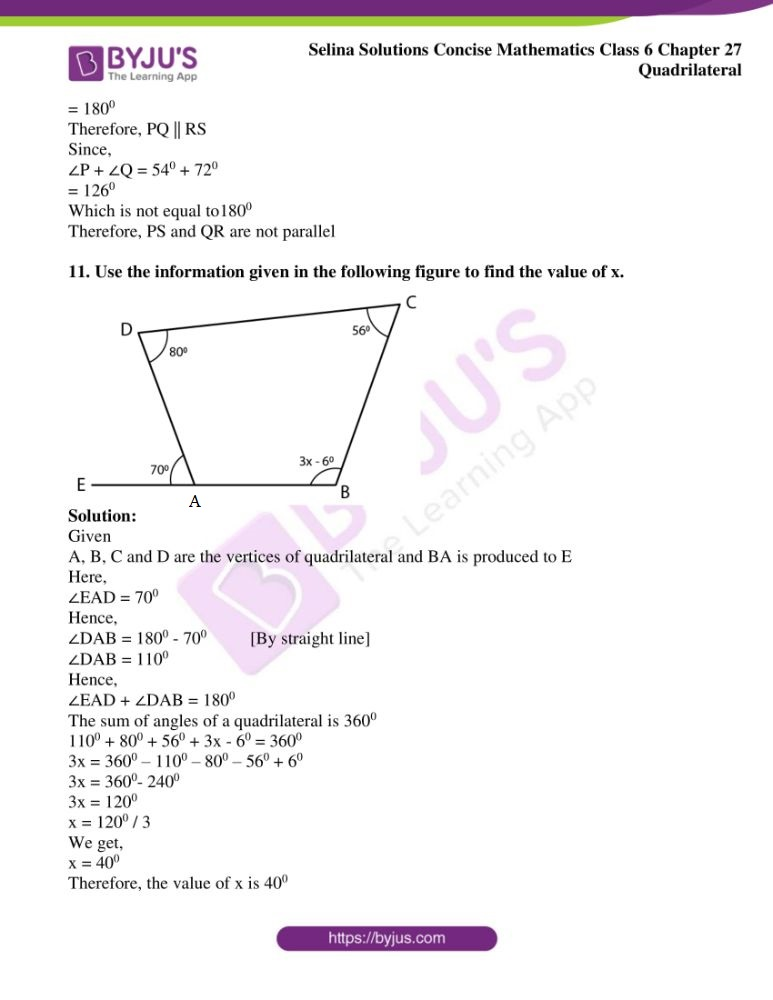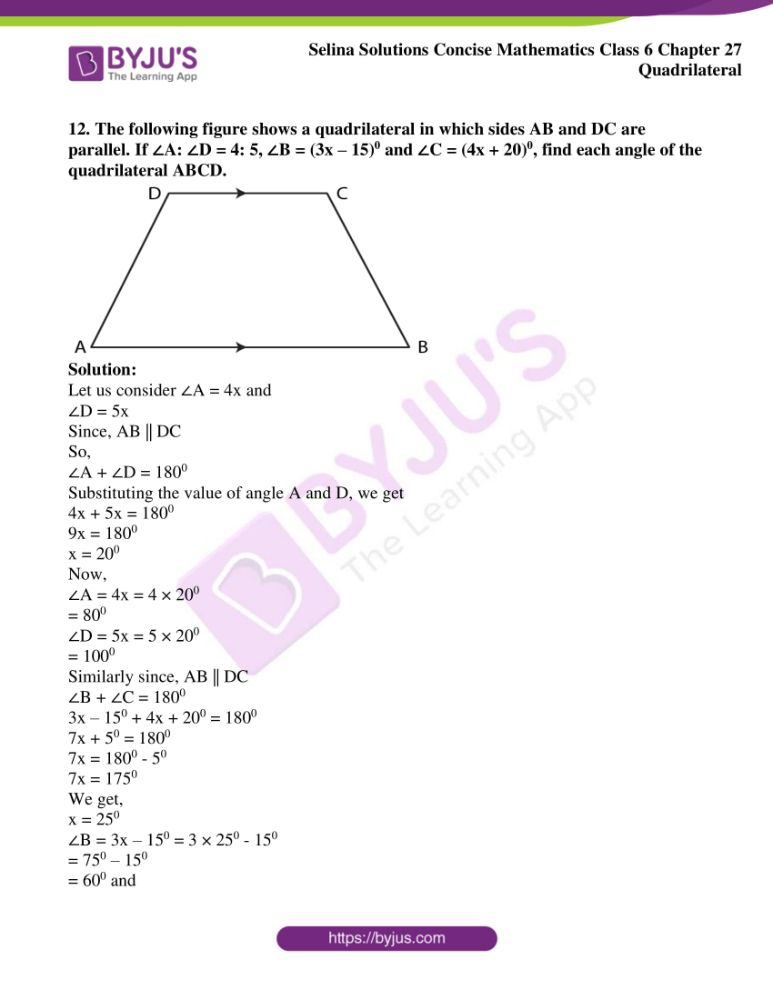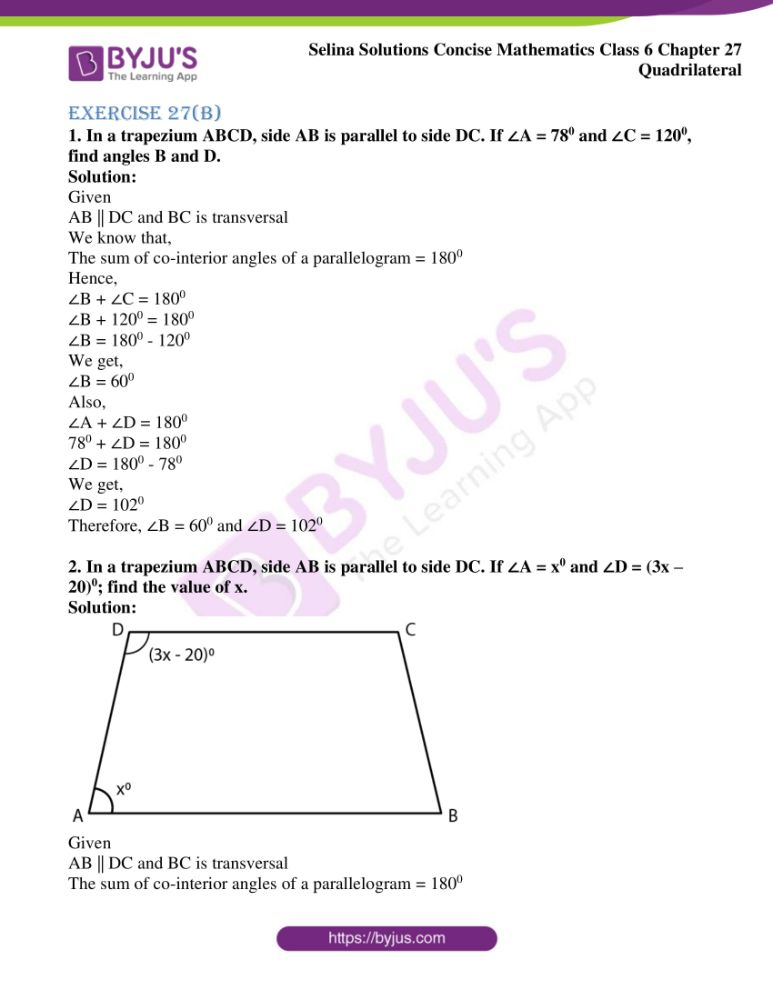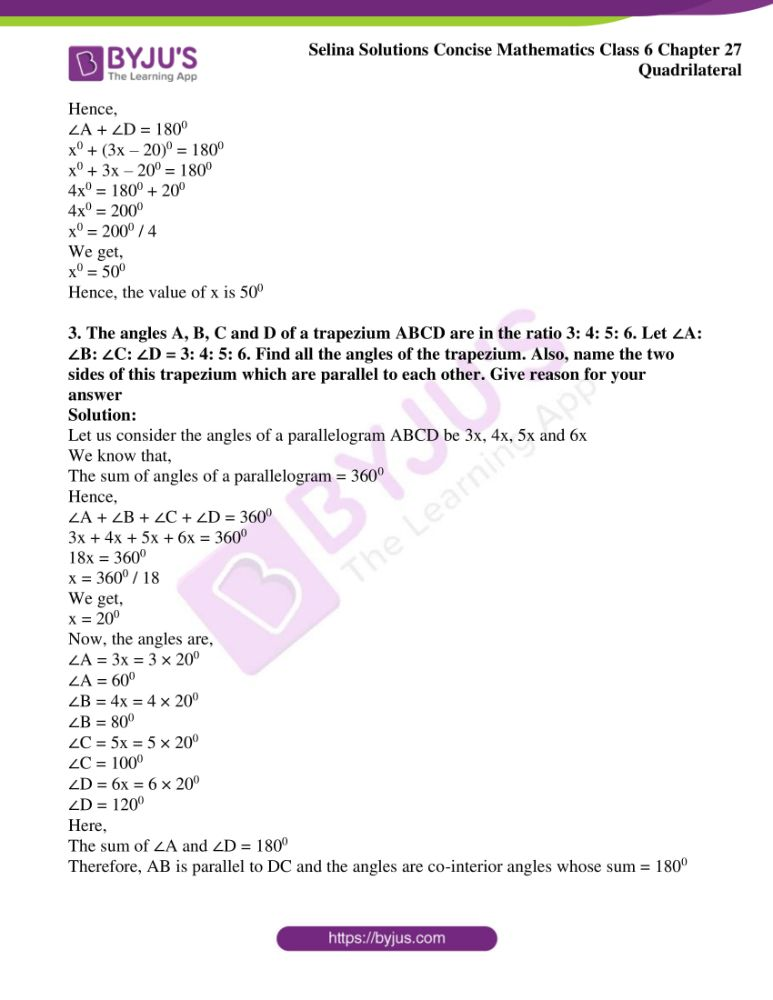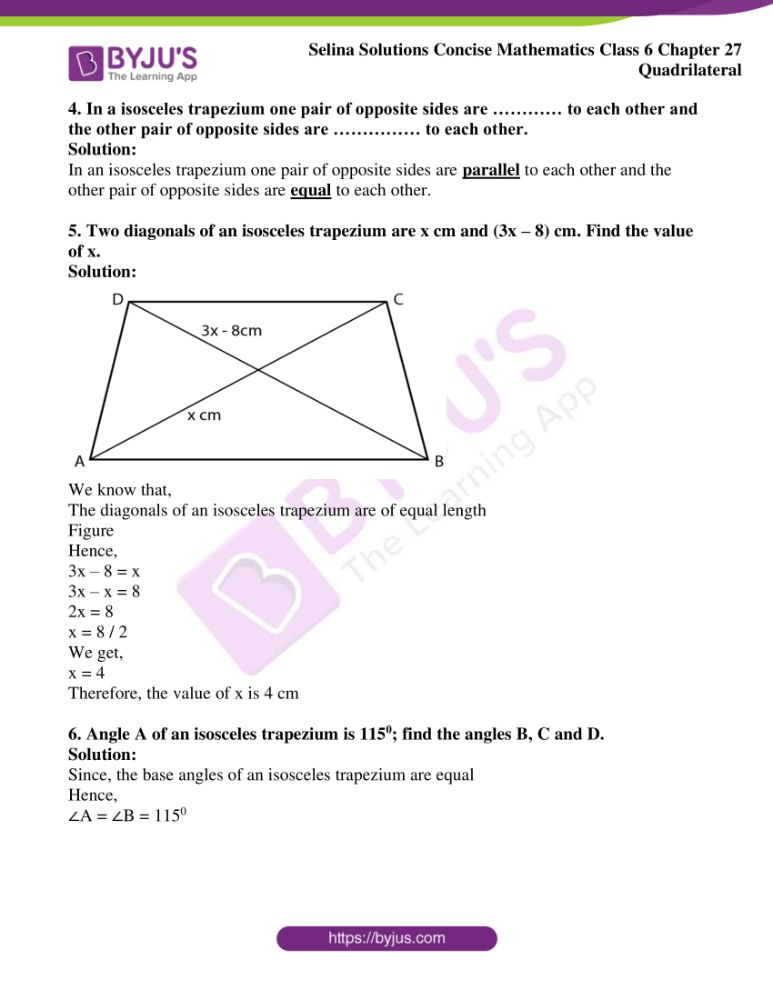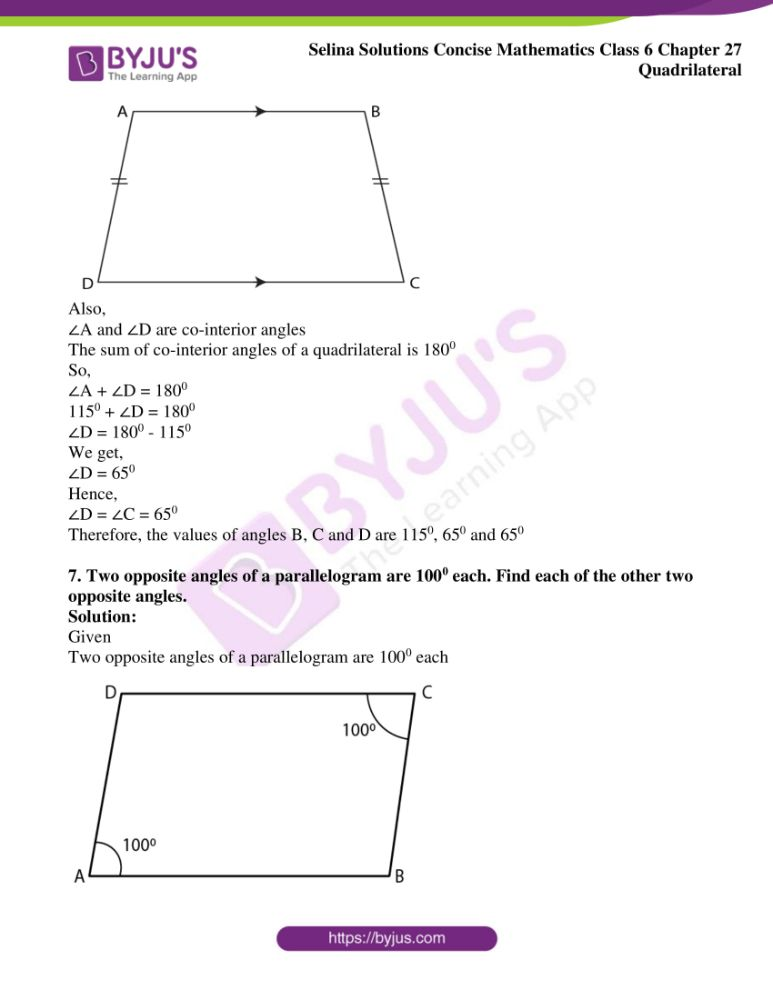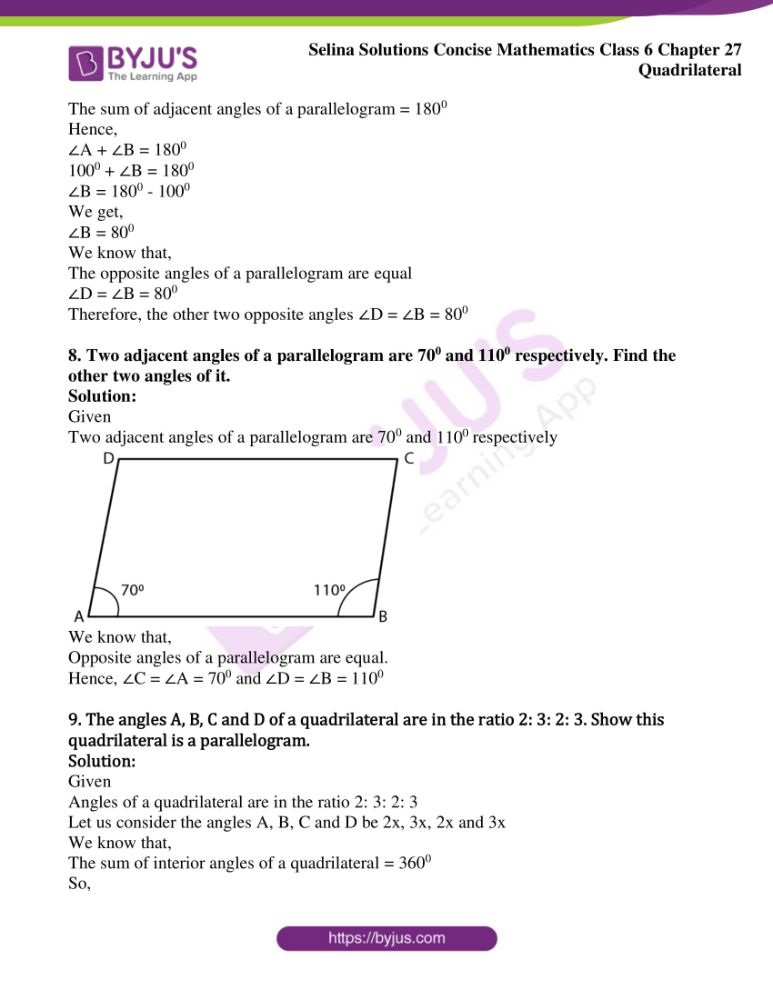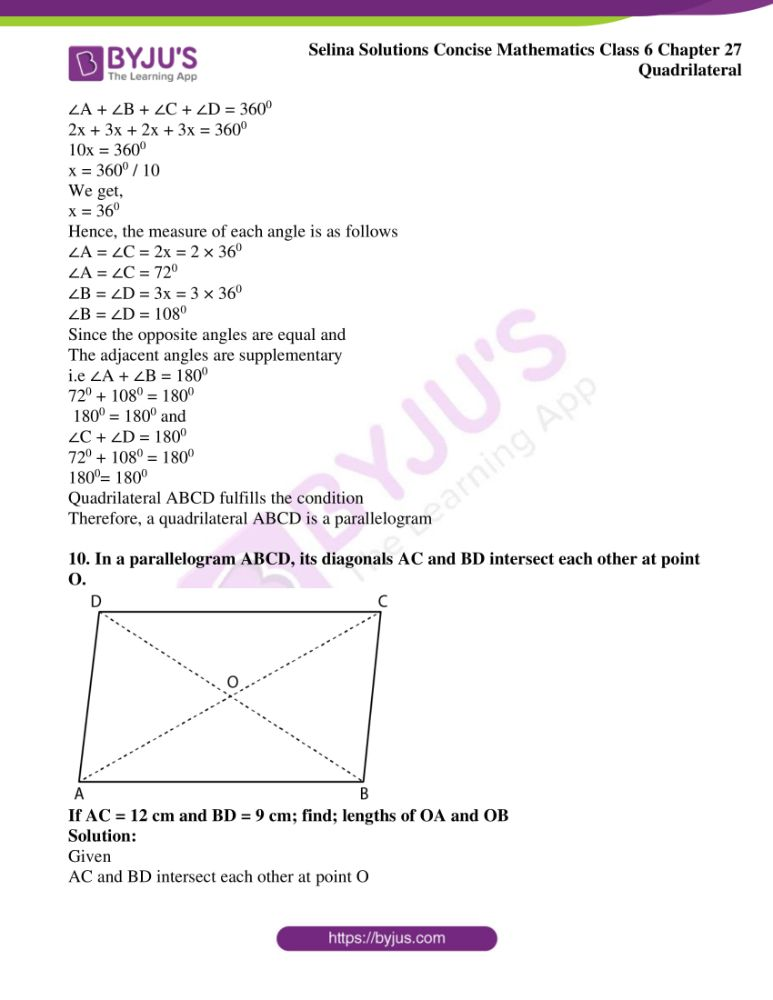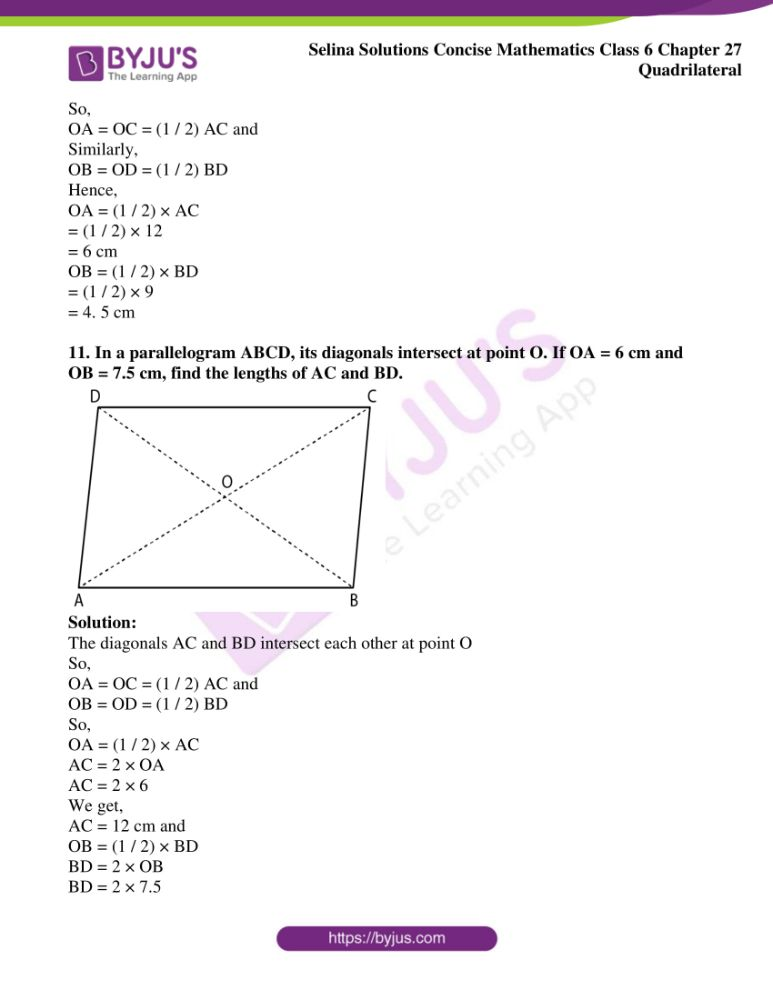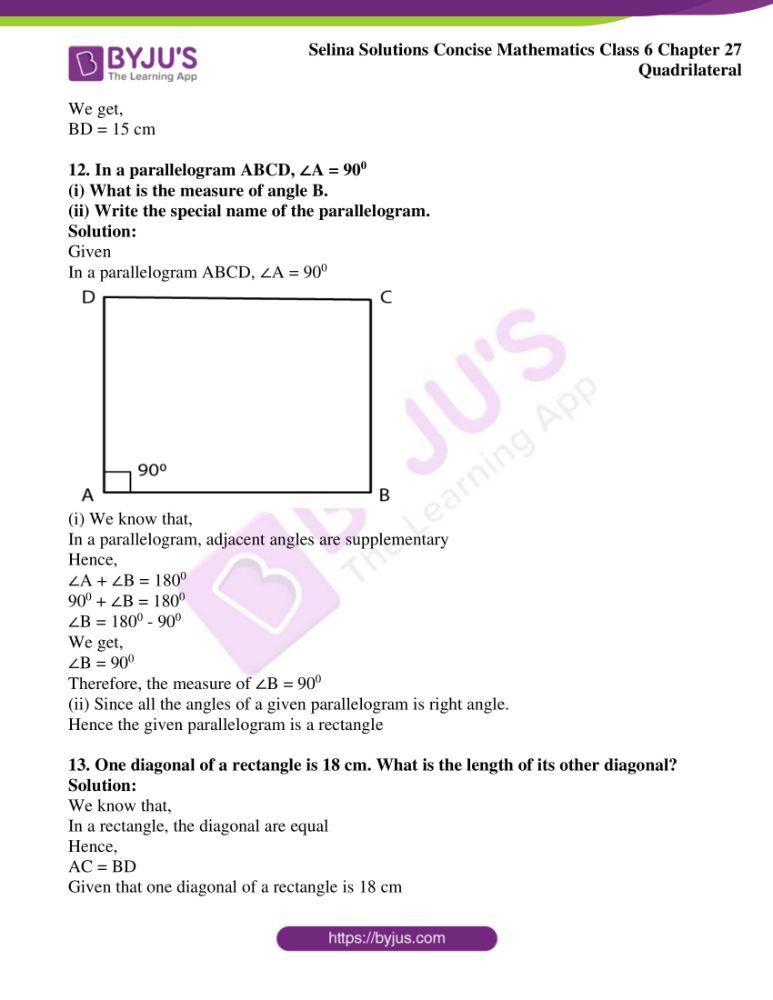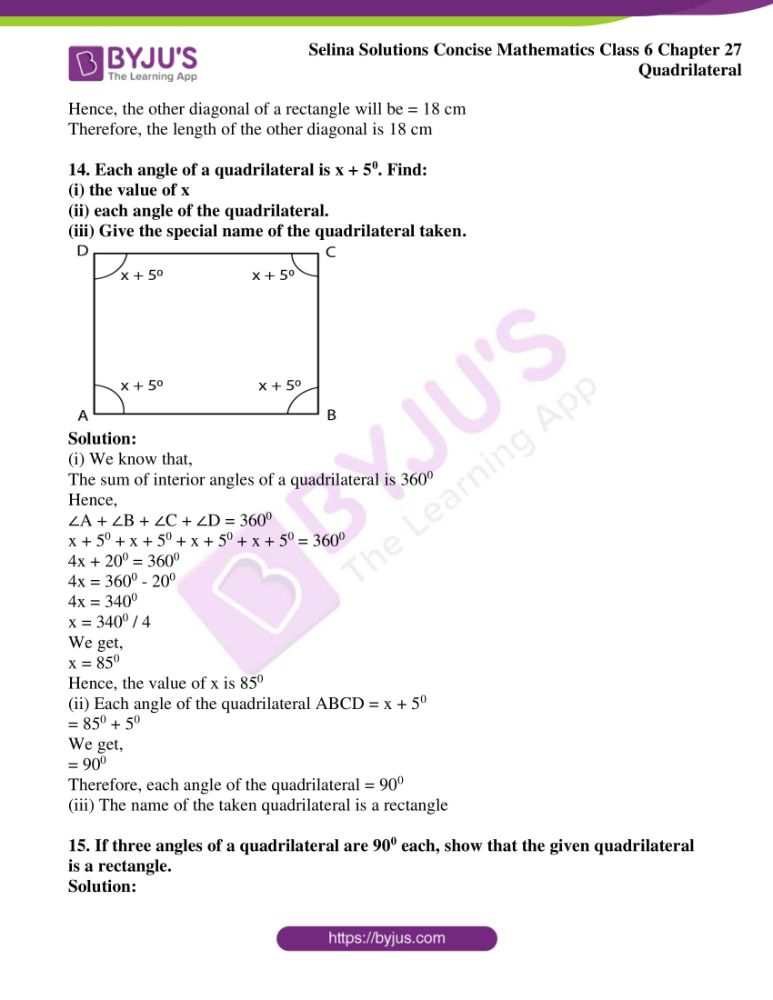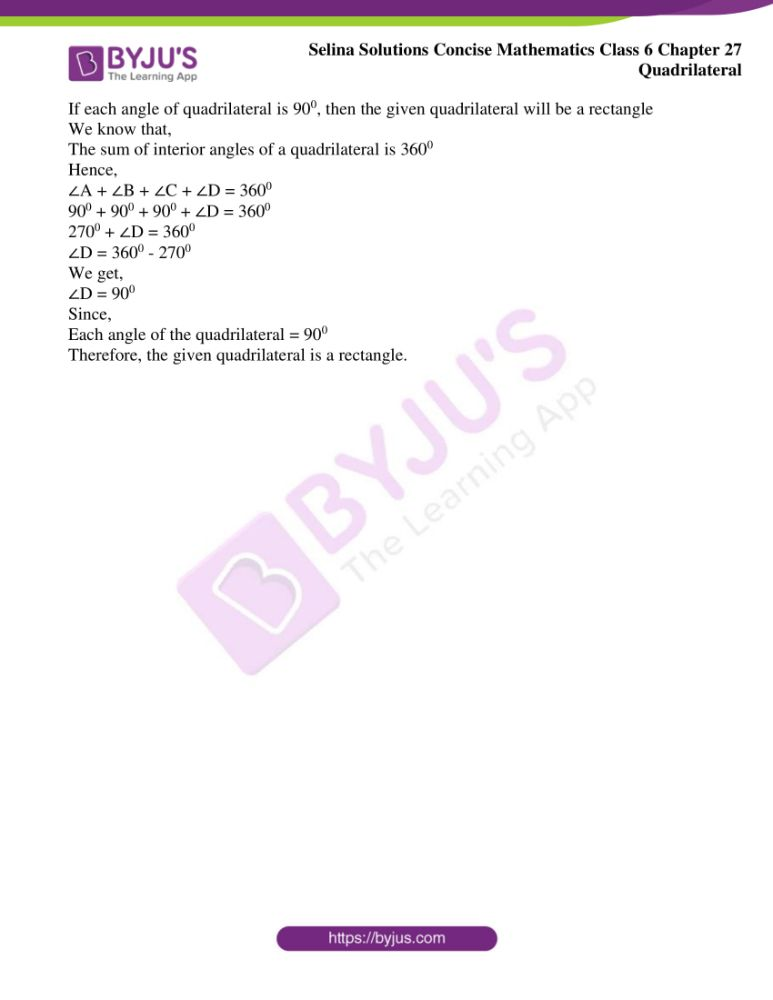### Exercises of Selina Solutions Concise Mathematics Class 6 Chapter 27: Quadrilateral

Exercise 27(A) Solutions

Exercise 27(B) Solutions

## Access Selina Solutions Concise Mathematics Class 6 Chapter 27 Quadrilateral

Exercise 27(A)

1. Two angles of a quadrilateral are 890 and 1130. If the other two angles are equal; find the equal angles.

Solution:

Let us consider the other angle as x0

As per the question, we have

890 + 1130 + x0 + x0 = 3600

2x0 = 3600 – 2020

2x0 = 158

x0 = 158 / 2

We get,

x = 790

Therefore, the other two equal angles are 790 each.

2. Two angles of a quadrilateral are 680 and 760. If the other two angles are in the ratio 5: 7; find the measure of each of them.

Solution:

Given

Two angles are 680 and 760

Let us consider the other two angles as 5x and 7x

Hence,

680 + 760 + 5x + 7x = 3600

12x + 1440 = 3600

12x = 3600 – 1440

12x = 2160

x = 2160 / 12

We get,

x = 180

Now, the other angles is calculated as below

5x = 5 × 180 = 900

7x = 7 × 180 = 1260

Therefore, the values of the other angles are 900 and 1260

3. Angles of a quadrilateral are (4x)0, 5(x+2)0, (7x – 20)0 and 6(x + 3)0. Find

(i) the value of x.

(ii) each angle of the quadrilateral.

Solution:

Given

(4x)0, 5(x + 2)0, (7x – 20)0 and 6(x + 3)0

We know that the sum of angles in a quadrilateral is 3600

Hence,

(4x)0 + 5(x + 2)0 + (7x – 20)0 + 6(x + 3)0 = 3600

4x + 5x + 100 + 7x – 200 + 6x + 180 = 3600

22x + 80 = 3600

22x = 3600 – 80

22x = 3520

x = 3520 / 22

We get,

x = 160

Hence, the value of x is 160

Therefore, the angles are,

(4x)0 = (4 × 16)0

= 640

5(x + 2)0 = 5(16 + 2)0

= 900

6(x + 3)0 = 6(16 + 3)0

= 1140

And,

(7x – 20)° = (7×16 – 20)°

= 92°

4. Use the information given in the following figure to find:

(i) x

(ii) ∠B and ∠C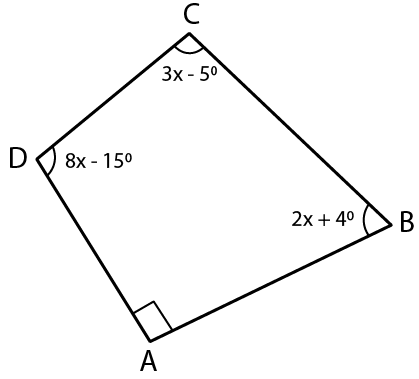Solution:

Here, given that,

∠A = 900

∠B = (2x + 4)0

∠C = (3x – 5)0

∠D = (8x – 15)0

We know that,

All the angles in a quadrilateral is 3600

So,

∠A + ∠B + ∠C + ∠D = 3600

900 + (2x + 4)0 + (3x – 5)0 + (8x – 15)0 = 3600

On further calculation, we get

900 + 2x + 40 + 3x – 50 + 8x – 150 = 3600

740 + 13x = 3600

13x = 3600 – 740

13x = 2860

x = 2860 / 13

We get,

x = 220

The value of x is 220

Now,

∠B = 2x + 4 = 2 × 220 + 4

= 480

∠C = 3x – 5 = 3 × 220 – 5

= 610

Therefore, ∠B = 480 and ∠C = 610

5. In quadrilateral ABCD, side AB is parallel to side DC. If ∠A: ∠D = 1: 2 and ∠C: ∠B = 4: 5

(i) Calculate each angle of the quadrilateral.

(ii) Assign a special name to quadrilateral ABCD.

Solution: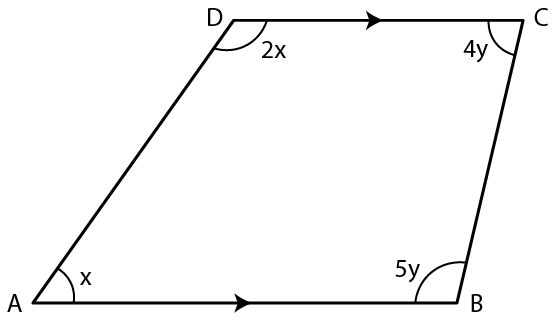Given

∠A: ∠D = 1: 2

Let us consider ∠A = x and ∠D = 2x

∠C: ∠B = 4: 5

Let us consider ∠C = 4y and ∠B = 5y

Also, given

AB || DC and the sum of opposite angles of quadrilateral is 1800

So,

∠A + ∠D = 1800

x + 2x = 1800

3x = 1800

We get,

x = 600

Therefore, ∠A = 600

∠D = 2x

= 2 × 600

= 1200

Therefore, ∠D = 1200

Now,

∠B + ∠C = 1800

5y + 4y = 1800

9y = 1800

We get,

y = 200

Now,

∠B = 5y = 5 × 200

= 1000

∠C = 4y = 4 × 200

= 800

Therefore, ∠A = 600; ∠B = 1000; ∠C = 800 and ∠D = 1200

6. From the following figure find:

(i) x,

(ii) ∠ABC,

(iii) ∠ACD.

Solution: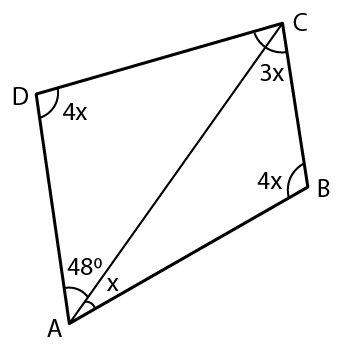(i)We know that,

In quadrilateral the sum of angles is equal to 3600

Hence,

x + 4x + 3x + 4x + 480 = 3600

12x = 3600 – 480

12x = 312

We get,

x = 260

Hence, the value of x is 260

(ii) ∠ABC = 4x

4 × 260 = 1040

Therefore, ∠ABC = 1040

(iii) ∠ACD = 1800 – 4x – 480

= 1800 – 4 × 260 – 480

= 1800 – 1040 – 480

We get,

= 280

Therefore, ∠ACD = 280

7. Given: In quadrilateral ABCD; ∠C = 640, ∠D = ∠C – 80; ∠A = 5(a + 2)0 and ∠B = 2(2a + 7)0.

Solution:

Given

∠C = 640

∠D = ∠C – 80

= 640 – 80

We get,

∠D = 560

∠A = 5 (a + 2)0

∠B = 2(2a + 7)0

We know that, sum of all the angles in a quadrilateral = 3600

So,

∠A + ∠B + ∠C + ∠D = 3600

5(a + 2)0 + 2(2a + 7)0 + 640 + 560 = 3600

On further calculation, we get

5a + 100 + 4a + 140 + 640 + 560 = 3600

9a + 1440 = 3600

9a = 3600 – 1440

9a = 2160

We get,

a = 240

∠A = 5(a + 2)

= 5(24 + 2)

We get,

= 1300

8. In the given figure

∠b = 2a + 15

And ∠c = 3a + 5; find the values of b and c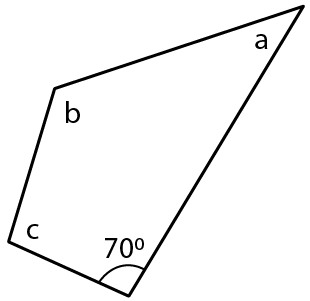Solution:

∠b = 2a + 15 and

∠c = 3a + 5

Sum of angles of a quadrilateral = 3600

700 + ∠a + ∠b + ∠c = 3600

700 + a + (2a + 15) + (3a + 5) = 3600

700 + a + 2a + 15 + 3a + 5 = 3600

6a + 900 = 3600

6a = 3600 – 900

6a = 2700

We get,

a = 450

Hence, ∠a = 450

b = 2a + 15 = 2 × 450 + 15

= 900 + 15

= 1050

c = 3a + 5 = 3 × 450 + 5

= 1350 + 5

= 1400

Therefore, ∠a = 450; ∠ b = 1050 and ∠c = 1400

9. Three angles of a quadrilateral are equal. If the fourth angle is 690; find the measure of equal angles.

Solution: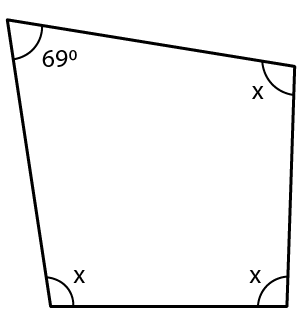Given that,

Three angles of a quadrilateral are equal

Let us consider each angle as x0

Hence,

x0 + x0 + x0 + 690 = 3600

3x = 3600 – 690

3x = 2910

x = 2910 / 3

We get,

x = 970

Therefore, the measure of all the equal angles is 970

10. In quadrilateral PQRS, ∠P: ∠Q : ∠R: ∠S = 3: 4: 6: 7.

Calculate each angle of the quadrilateral and then prove that PQ and SR are parallel to each other. Is PS also parallel to QR?

Solution: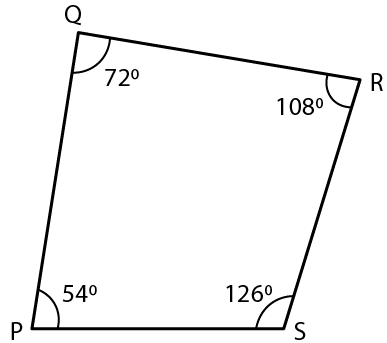Given

∠P: ∠Q: ∠R: ∠S = 3: 4: 6: 7

Let ∠P = 3x

∠Q = 4x

∠R = 6x and

∠S = 7x

Hence,

∠P + ∠Q + ∠R + ∠S = 3600

3x + 4x + 6x + 7x = 3600

20x = 3600

x = 3600 / 20

We get,

x = 180

So,

∠P = 3x = 3 × 180

= 540

∠Q = 4x = 4 × 180

= 720

∠R = 6x = 6 × 180

= 1080

∠S = 7x = 7 × 180

= 1260

∠Q + ∠R = 720 + 1080

= 1800 and

∠P + ∠S = 540 + 1260

= 1800

Therefore, PQ || RS

Since,

∠P + ∠Q = 540 + 720

= 1260

Which is not equal to1800

Therefore, PS and QR are not parallel

11. Use the information given in the following figure to find the value of x.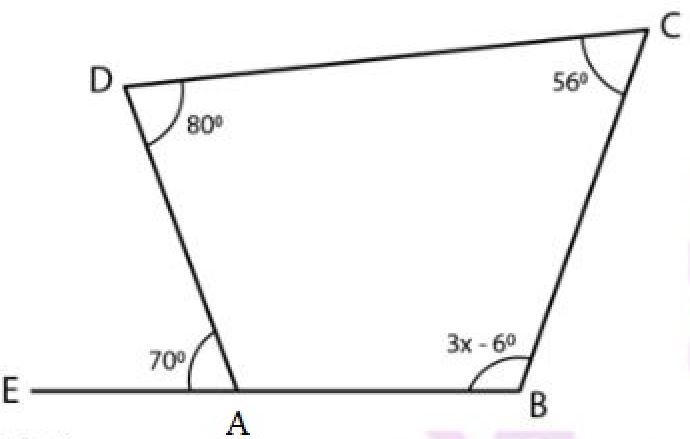Solution:

Given

A, B, C and D are the vertices of quadrilateral and BA is produced to E

Here,

Hence,

∠DAB = 1800 – 700 [By straight line]

∠DAB = 1100

Hence,

The sum of angles of a quadrilateral is 3600

1100 + 800 + 560 + 3x – 60 = 3600

3x = 3600 – 1100 – 800 – 560 + 60

3x = 3600– 2400

3x = 1200

x = 1200 / 3

We get,

x = 400

Therefore, the value of x is 400

12. The following figure shows a quadrilateral in which sides AB and DC are parallel. If ∠A: ∠D = 4: 5, ∠B = (3x – 15)0 and ∠C = (4x + 20)0, find each angle of the quadrilateral ABCD.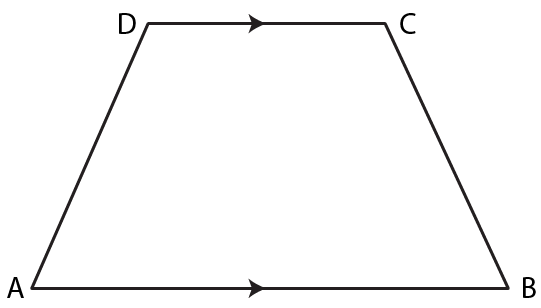Solution:

Let us consider ∠A = 4x and

∠D = 5x

Since, AB || DC

So,

∠A + ∠D = 1800

Substituting the value of angle A and D, we get

4x + 5x = 1800

9x = 1800

x = 200

Now,

∠A = 4x = 4 × 200

= 800

∠D = 5x = 5 × 200

= 1000

Similarly since, AB || DC

∠B + ∠C = 1800

3x – 150 + 4x + 200 = 1800

7x + 50 = 1800

7x = 1800 – 50

7x = 1750

We get,

x = 250

∠B = 3x – 150 = 3 × 250 – 150

= 750 – 150

= 600 and

∠C = 4x + 200 = 4 × 250 + 200

= 1000 + 200

= 1200

Exercise 27(B)

1. In a trapezium ABCD, side AB is parallel to side DC. If ∠A = 780 and ∠C = 1200, find angles B and D.

Solution:

Given

AB || DC and BC is transversal

We know that,

The sum of co-interior angles of a parallelogram = 1800

Hence,

∠B + ∠C = 1800

∠B + 1200 = 1800

∠B = 1800 – 1200

We get,

∠B = 600

Also,

∠A + ∠D = 1800

780 + ∠D = 1800

∠D = 1800 – 780

We get,

∠D = 1020

Therefore, ∠B = 600 and ∠D = 1020

2. In a trapezium ABCD, side AB is parallel to side DC. If ∠A = x0 and ∠D = (3x – 20)0; find the value of x.

Solution: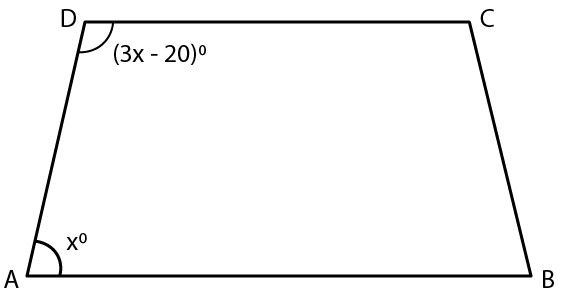Given

AB || DC and BC is transversal

The sum of co-interior angles of a parallelogram = 1800

Hence,

∠A + ∠D = 1800

x0 + (3x – 20)0 = 1800

x0 + 3x – 200 = 1800

4x0 = 1800 + 200

4x0 = 2000

x0 = 2000 / 4

We get,

x0 = 500

Hence, the value of x is 500

3. The angles A, B, C and D of a trapezium ABCD are in the ratio 3: 4: 5: 6. Let ∠A: ∠B: ∠C: ∠D = 3: 4: 5: 6. Find all the angles of the trapezium. Also, name the two sides of this trapezium which are parallel to each other. Give reason for your answer

Solution:

Let us consider the angles of a parallelogram ABCD be 3x, 4x, 5x and 6x

We know that,

The sum of angles of a parallelogram = 3600

Hence,

∠A + ∠B + ∠C + ∠D = 3600

3x + 4x + 5x + 6x = 3600

18x = 3600

x = 3600 / 18

We get,

x = 200

Now, the angles are,

∠A = 3x = 3 × 200

∠A = 600

∠B = 4x = 4 × 200

∠B = 800

∠C = 5x = 5 × 200

∠C = 1000

∠D = 6x = 6 × 200

∠D = 1200

Here,

The sum of ∠A and ∠D = 1800

Therefore, AB is parallel to DC and the angles are co-interior angles whose sum = 1800

4. In a isosceles trapezium one pair of opposite sides are ………… to each other and the other pair of opposite sides are …………… to each other.

Solution:

In an isosceles trapezium one pair of opposite sides are parallel to each other and the other pair of opposite sides are equal to each other.

5. Two diagonals of an isosceles trapezium are x cm and (3x – 8) cm. Find the value of x.

Solution: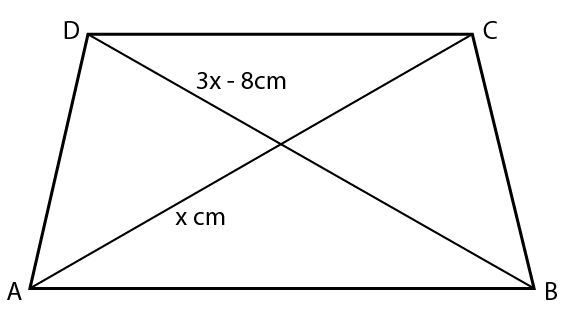We know that,

The diagonals of an isosceles trapezium are of equal length

Figure

Hence,

3x – 8 = x

3x – x = 8

2x = 8

x = 8 / 2

We get,

x = 4

Therefore, the value of x is 4 cm

6. Angle A of an isosceles trapezium is 1150; find the angles B, C and D.

Solution:

Since, the base angles of an isosceles trapezium are equal

Hence,

∠A = ∠B = 1150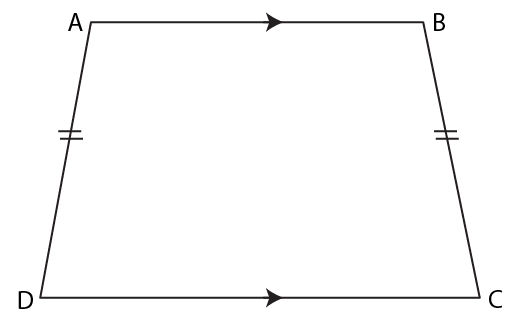Also,

∠A and ∠D are co-interior angles

The sum of co-interior angles of a quadrilateral is 1800

So,

∠A + ∠D = 1800

1150 + ∠D = 1800

∠D = 1800 – 1150

We get,

∠D = 650

Hence,

∠D = ∠C = 650

Therefore, the values of angles B, C and D are 1150, 650 and 650

7. Two opposite angles of a parallelogram are 1000 each. Find each of the other two opposite angles.

Solution:

Given

Two opposite angles of a parallelogram are 1000 each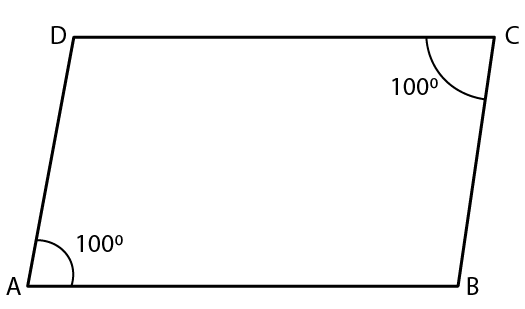The sum of adjacent angles of a parallelogram = 1800

Hence,

∠A + ∠B = 1800

1000 + ∠B = 1800

∠B = 1800 – 1000

We get,

∠B = 800

We know that,

The opposite angles of a parallelogram are equal

∠D = ∠B = 800

Therefore, the other two opposite angles ∠D = ∠B = 800

8. Two adjacent angles of a parallelogram are 700 and 1100 respectively. Find the other two angles of it.

Solution:

Given

Two adjacent angles of a parallelogram are 700 and 1100 respectively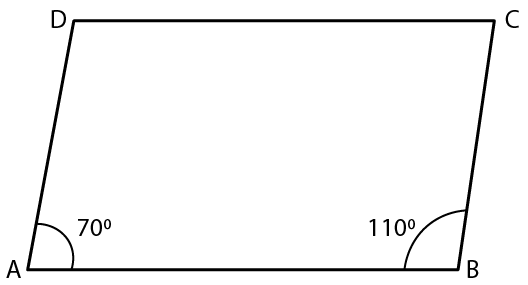We know that,

Opposite angles of a parallelogram are equal.

Hence, ∠C = ∠A = 700 and ∠D = ∠B = 1100

9. The angles A, B, C and D of a quadrilateral are in the ratio 2: 3: 2: 3. Show this quadrilateral is a parallelogram.

Solution:

Given

Angles of a quadrilateral are in the ratio 2: 3: 2: 3

Let us consider the angles A, B, C and D be 2x, 3x, 2x and 3x

We know that,

The sum of interior angles of a quadrilateral = 3600

So,

∠A + ∠B + ∠C + ∠D = 3600

2x + 3x + 2x + 3x = 3600

10x = 3600

x = 3600 / 10

We get,

x = 360

Hence, the measure of each angle is as follows

∠A = ∠C = 2x = 2 × 360

∠A = ∠C = 720

∠B = ∠D = 3x = 3 × 360

∠B = ∠D = 1080

Since the opposite angles are equal and

i.e ∠A + ∠B = 1800

720 + 1080 = 1800

1800 = 1800 and

∠C + ∠D = 1800

720 + 1080 = 1800

1800= 1800

Therefore, a quadrilateral ABCD is a parallelogram

10. In a parallelogram ABCD, its diagonals AC and BD intersect each other at point O.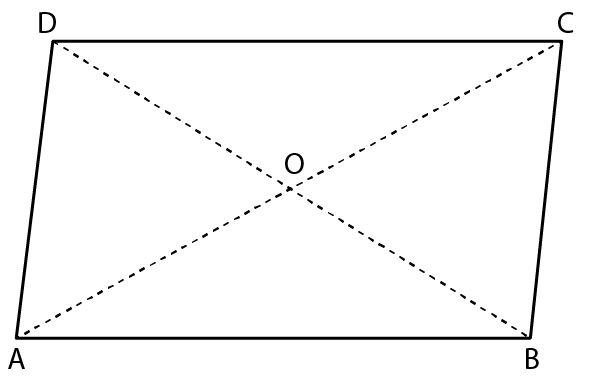If AC = 12 cm and BD = 9 cm; find; lengths of OA and OB

Solution:

Given

AC and BD intersect each other at point O

So,

OA = OC = (1 / 2) AC and

Similarly,

OB = OD = (1 / 2) BD

Hence,

OA = (1 / 2) × AC

= (1 / 2) × 12

= 6 cm

OB = (1 / 2) × BD

= (1 / 2) × 9

= 4. 5 cm

11. In a parallelogram ABCD, its diagonals intersect at point O. If OA = 6 cm and OB = 7.5 cm, find the lengths of AC and BD.Solution:

The diagonals AC and BD intersect each other at point O

So,

OA = OC = (1 / 2) AC and

OB = OD = (1 / 2) BD

So,

OA = (1 / 2) × AC

AC = 2 × OA

AC = 2 × 6

We get,

AC = 12 cm and

OB = (1 / 2) × BD

BD = 2 × OB

BD = 2 × 7.5

We get,

BD = 15 cm

12. In a parallelogram ABCD, ∠A = 900

(i) What is the measure of angle B.

(ii) Write the special name of the parallelogram.

Solution:

Given

In a parallelogram ABCD, ∠A = 900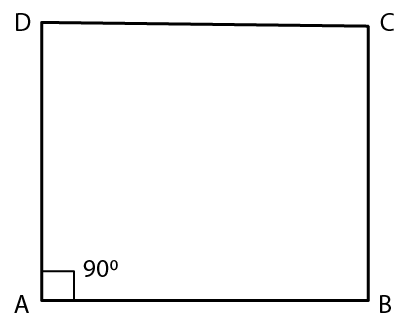(i) We know that,

In a parallelogram, adjacent angles are supplementary

Hence,

∠A + ∠B = 1800

900 + ∠B = 1800

∠B = 1800 – 900

We get,

∠B = 900

Therefore, the measure of ∠B = 900

(ii) Since all the angles of a given parallelogram is right angle.

Hence the given parallelogram is a rectangle

13. One diagonal of a rectangle is 18 cm. What is the length of its other diagonal?

Solution:

We know that,

In a rectangle, the diagonal are equal

Hence,

AC = BD

Given that one diagonal of a rectangle is 18 cm

Hence, the other diagonal of a rectangle will be = 18 cm

Therefore, the length of the other diagonal is 18 cm

14. Each angle of a quadrilateral is x + 50. Find:

(i) the value of x

(ii) each angle of the quadrilateral.

(iii) Give the special name of the quadrilateral taken.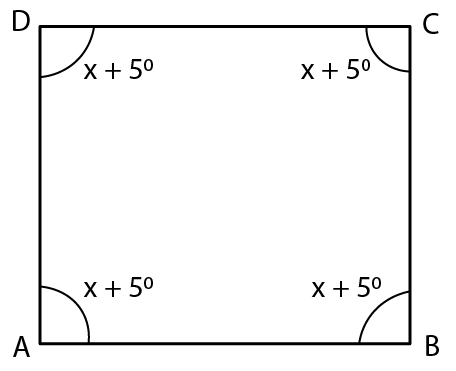Solution:

(i) We know that,

The sum of interior angles of a quadrilateral is 3600

Hence,

∠A + ∠B + ∠C + ∠D = 3600

x + 50 + x + 50 + x + 50 + x + 50 = 3600

4x + 200 = 3600

4x = 3600 – 200

4x = 3400

x = 3400 / 4

We get,

x = 850

Hence, the value of x is 850

(ii) Each angle of the quadrilateral ABCD = x + 50

= 850 + 50

We get,

= 900

Therefore, each angle of the quadrilateral = 900

(iii) The name of the taken quadrilateral is a rectangle

15. If three angles of a quadrilateral are 900 each, show that the given quadrilateral is a rectangle.

Solution:

If each angle of quadrilateral is 900, then the given quadrilateral will be a rectangle

We know that,

The sum of interior angles of a quadrilateral is 3600

Hence,

∠A + ∠B + ∠C + ∠D = 3600

900 + 900 + 900 + ∠D = 3600

2700 + ∠D = 3600

∠D = 3600 – 2700

We get,

∠D = 900

Since,

Each angle of the quadrilateral = 900

Therefore, the given quadrilateral is a rectangle.